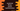# Binary search implementation in JavaScript## Binary search implementation in JavaScript:

Binary search is used to search for an element in a sorted array. It is faster than linear search and its time complexity is O(logN). The time complexity of Linear search is O(N).

In this post, I will show you how to implement Binary search in JavaScript. We will learn how to write it in recursive and iterative methods.

The program will take an array of sorted numbers and the number to search as inputs. Before we start writing the program, let me quickly explain how binary search works.

### How binary search works:

Binary Search uses divide and conquer approach to search for a number in a sorted array.

• It finds the mid-point in the array and compares the search value with the array value.
• If both are equal, the search is completed. Return the mid index of the array.
• If both are not equal, check if the search value is smaller or bigger than the mid value.
• Since, the search is on a sorted array, if the search value is bigger than the mid value, we can continue the search on the right side of the array, i.e. in between mid + 1 to end of the array. Similarly, if the search value is smaller than the mid value, we can continue the search on the left side of the array, i.e. in between 0 to mid - 1.
• Based on the comparison, continue the search.
• If at some point, the start index is smaller than the end index, return -1, i.e. the number is not found in that array.

Now, let’s write it down in code.

### JavaScript Binary Search implementation (Iterative way):

Let’s write down the program in iterative way:

``````function binarySearch(arr, n) {
let startIndex = 0;
let endIndex = arr.length - 1;

while (startIndex <= endIndex) {
let midIndex = Math.floor((startIndex + endIndex) / 2);

if (arr[midIndex] === n) {
return midIndex;
}

if (arr[midIndex] < n) {
startIndex = midIndex + 1;
} else {
endIndex = midIndex - 1;
}
}

return -1;
}

arr = [0, 1, 2, 3, 4, 5];
testArrayElements = [-1, 0, 1, 2, 3, 4, 5, 6];

testArrayElements.forEach((e) =>
console.log(`\${e} => \${binarySearch(arr, e)}`)
);``````

Here,

• binarySearch method uses binary search to search n in the sorted array arr.
• Initially, it defines the start index as 0 and end index as the last index of the array.
• The while loop runs until the start index is smaller than or equal to the end index.
• Inside the loop, it finds the middle index.
• If the mid-value is equal to n, it returns the middle index.
• Else, if n is greater than the mid-value, it updates the start index. Similarly, if n is smaller than the mid-value, it updates the end index.
• It returns -1 if the while loop ends and if the number is not found by the while loop.
• We are using a forEach loop to search each numbers of testArrayElements in the arr array.

If you run this program, it will print output as like below:

``````-1 => -1
0 => 0
1 => 1
2 => 2
3 => 3
4 => 4
5 => 5
6 => -1``````

As you can see, all numbers of testArrayElements are found in arr except -1 and 6. It returns -1 for these two numbers.

### JavaScript Binary Search implementation (Recursive way):

We can write the above program recursively. A recursive method calls itself again and again to get the final result. Let’s write the program:

``````function binarySearch(arr, n, startIndex, endIndex) {
if (startIndex > endIndex) {
return -1;
}

let midIndex = Math.floor((startIndex + endIndex) / 2);

if (arr[midIndex] === n) {
return midIndex;
}

if (arr[midIndex] > n) {
return binarySearch(arr, n, startIndex, midIndex - 1);
} else {
return binarySearch(arr, n, midIndex + 1, endIndex);
}
}

arr = [0, 1, 2, 3, 4, 5];
testArrayElements = [-1, 0, 1, 2, 3, 4, 5, 6];

testArrayElements.forEach((e) =>
console.log(`\${e} => \${binarySearch(arr, e, 0, arr.length - 1)}`)
);``````
• The binarySearch method is changed to a recursive method.
• It takes the array, search value, start index and end index as the parameters. The start index is passed as 0 and the end index is passed as array size - 1 to this method.
• If the start index is greater than the end index, it returns -1.
• Similar to the previous program, it finds the middle index.
• If the element in the middle index is the search value, it returns that index.
• Else, it compares that number with the search value. Based on this comparison, it calls itself again recursively to get the final position.

There should always be an endpoint for recursive functions, i.e. it should stop at some point. We can’t keep it running for an infinite time. The first if statement works like that. It makes sure that the function returns -1 if no value is found.

We are using the same test array and test values. If you run this program, it will print output as like below:

``````-1 => -1
0 => 0
1 => 1
2 => 2
3 => 3
4 => 4
5 => 5
6 => -1``````